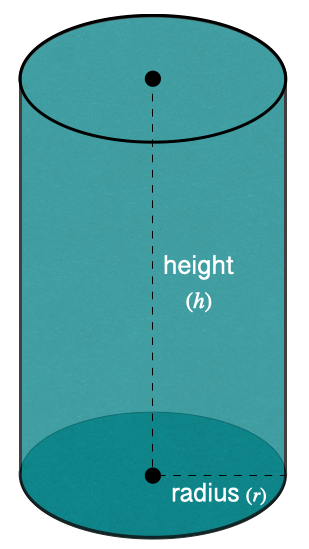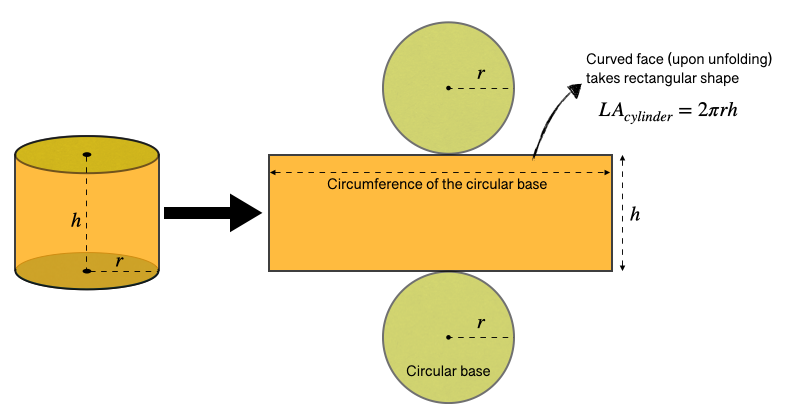407 493 6601

## Introduction

• A cylinder is a three-dimensional solid with two parallel circular bases connected by a curved face.
• A cylinder is analogous to a prism but with circular bases.• The height $\left(h\right)$ of a right circular cylinder is the measure of the line segment joining the center of two bases.
• A cylinder's size depends on the base's radius and height.
• Area of the base: $B={\mathrm{\pi r}}^{2}$

## Volume of a Cylinder

• A cylinder's volume (capacity) is the amount of space it occupies in a three-dimensional plane.
• A cylinder's volume is measured in cubic units.
• If we know the area of the base $\left(B\right)$ and height $\left(h\right)$ of the right circular cylinder, its volume can be calculated using the formula mentioned below.

$Volum{e}_{cylinder}=B×h$

• If we know the radius of the base $\left(r\right)$ and height $h$ of the right circular cylinder, its volume can be calculated using the formula mentioned below.

$Volum{e}_{cylinder}={\mathrm{\pi r}}^{2}h$

## Surface Area of a Cylinder

• There are two types of surface areas associated with a right circular cylinder.

1. Lateral Area: It is the region occupied by the curved surface of the right circular cylinder.

$L{A}_{cylinder}=2\pi rh$, where $r=$radius of the base, and $h=$height2. Total Area: It is the total area occupied by a right circular cylinder, including the area of the two bases.

$T{A}_{cylinder}=L{A}_{cylinder}+2B$

$=2\mathrm{\pi r}\left(h+\mathrm{r}\right)$, where $B=$area of the base

## Solved Examples

Question 1: Find the volume of a cylinder with a radius of 4 inches and a height of 6 inches.

Solution: ${V}_{cylinder}={\mathrm{\pi r}}^{2}\mathrm{h}$

$=\mathrm{\pi }×\left({6}^{2}\right)×4$

Question 2: Find the total surface area of a cylinder with a radius of 5 cm and a height of 10 cm.

Solution: $T{A}_{cylinder}=2\mathrm{\pi r}\left(h+r\right)$

## Cheat Sheet

• Area of the base, $B={\mathrm{\pi r}}^{2}$
• $Volum{e}_{cylinder}={\mathrm{\pi r}}^{2}h$
• Lateral Surface Area, $L{A}_{cylinder}=2\mathrm{\pi r}h$
• Total Surface Area, $T{A}_{cylinder}=L{A}_{cylinder}+2B$$=2\mathrm{\pi r}\left(h+\mathrm{r}\right)$

## Blunder Areas

• The lateral surface area and curved surface are the same.
• Do not forget to multiply the area of the base (B) by two in the formula of the total surface area of a cylinder, i.e. .